## Appendix A: Boiler Calculations

This appendix is included to enhance the appreciation of the fundamentals and to sim­plify basic process calculations.

• Boilers encounter different steaming conditions using a variety of fuels, and are calculation-intensive. All boiler users are required to perform the peripheral or process calculations. For that reason they are included here.

• The sizing calculations of various boiler parts that require proprietary data and the coded strength calculations for various pressure parts (PPs), are not included because they are of interest only to boiler designers.

• A small nonreheat industrial stoker-fired boiler for coal firing was selected (see Table A1) to keep the calculations simple, so that the methodology is well understood.

• When calculations are done on different systems of units, some marginal differ­ences are inevitable in the end results because of rounding of figures and small differences in conversions. These are best ignored.

• The calculations are kept simple. Complex calculations may be required only by the boilermakers.

• Calculation methods, safety margins, range of conditions, etc., vary with the boil­ermakers, boiler sizes, and clients/consultants. Other than in coded calculations, there are slight variations in sizing and design practices.

• Most calculations are performed in all three systems, namely, FPS, metric, and SI units to make them understandable to all.

Combustion Calculations Calculation A.1.1.1

To calculate heat duty of the boiler on gross steam generation. Refer to Table A2.

Please refer to the steam tables for values. In this example, the gross evaporation from the boiler is considered as 68 tph. This is different from net evaporation of 68 tph when, like in many process boilers, the boiler has to provide steam for deaerator. The gross steam gen­eration in such a case would be 68 tph plus heat to deaerator. The next example calculates the heat duty on net evaporation basis.

Calculation A.1.1.2

To calculate heat duty on net evaporation.

Net evaporation is gross evaporation minus steam for deaeration.

 In SI Units In Metric Units In FPS Units Steaming conditions Evaporation (MCR) 18.895 kg/s 68 tph 150,000 lb/h Superheater outlet pressure (SOP) 70 bar 70.38 kg/cm2 g 1,000 psig Superheater outlet temperature (SOT) 400°C 400°C 752°F Feed water temperature (FWT) 105°C 105°C 221°F Ambient conditions Temperature 77°F 25°C Relative humidity 60% 60% Altitude 1000 ft 305 m Coal specifications: proximate analysis A% M% VM% FC% Total S Gross calorific value 24.49 MJ/kg 15.0 10.0 30.0 45.0 100.0 1.0 5,850 kcal/kg 10,530 Btu/lb

TABLE A2

Heat Duty Calculation (Calculation A.1.1.1)

In SI Units In MKS Units In FPS Units

Pressure Temperature Enthalpy Pressure Temperature Enthalpy Pressure Temperature Enthalpy

(Bar) (°C) (kJ/kg) (kg/cm2 g) (°C) (kcal/kg) (psig) (°F) (Btu/lb)

 SOP 70 400 3161.2 70.38 400 756 1000 752 1359 FWT — 105 440.2 — 105 105.2 — 221 189 Heat to 2721.0 650 1170 Steam Steam flow 18.895 kg/s 68.02 tph 150,000 lb/h At MCR Heat duty 51.42 44.26 175.5 M = Heat to MWth M kcal/h Btu/h

Steam x

MCR

(maximum

Continuous

Rating)

For deaerator, let us assume the inlet temperature of condensate as 85°C (185°F). Heat balance across the deaerator gives the steam for deaeration. In Figure A1,

Heat in condensate + heating steam = heat in feed water (FW)

68,020 X 85 + s X 756 = (68,020 + s) X 105.2

Which gives the value of

_ 68,020 X (105.2 — 85)

2287kg/h

756 -105.2

Revised steam flow = 70,307 kg/h, which is higher by 3.36%. The revised heat duty will also be higher by the same proportion.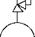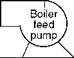Deaerator storage tank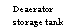E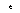FIGURE A1

Deaerator and boiler feed pump.

Calculation A.1.1.3

To calculate the ultimate analysis (UA) from proximate analysis (PA). Refer Tables A3 and A4.

Step 1. Calculate daf volatile matter (VM) and fixed carbon (FC) as given in Table A3. Step 2. Refer to Section 2.6.8.I. for calculating the UA from PA.

% daf C + S = 60.0 + 0.9(40.0 — 14) = 83.40%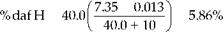% daf N = 2.1 — 0.012 X 40.0 = 1.62%

% daf O = by difference = 9.62%

Step 3. Work out the actual UA from daf basis by multiplying the figures with 1 — 0.25 = 0.75 as calculated in Table A4.

S needs to be deleted from C.

Calculation A.1.1.4

To check for gross calorific value (GCV) using Dulong’s formula given in Section 3.2.1.1.3. GCV in kcal/kg = (80.8 X 61.56) + 344.6[4.02 — (7.21/8)] + 22.5 X 1.00 = 6071 GCV in Btu/lb = (145.44 X 61.56) + 620.28[4.02 — (7.21/8)] + 40.5 X 1.00 = 10,928

The GCV is higher by —3.8% from the starting figure, which is within the acceptable tolerance limits.

TABLE A3

Proximate Analysis of Coal on daf Basis (Calculation A.1.1.3)

PA daf PA

 A% 15.0 — M% 10.0 — VM% 30.0 30/(1 — 0.25) = 40.0 FC% 45.0 45/(1 — 0.25) = 60.0 Total 100.0 100.0 S 1.0 GCV 24.49 MJ/kg 5850 kcal/kg 10,530 Btu/lb

TABLE A4

Calculation of Ultimate Analysis from Proximate Analysis (Calculation A.1.1.3)

 Element Percentage S Adjustment C 83.4 X 0.75 ; = 62.56 61.56 H 5.36 X 0.75 = 4.02 4.02 O 9.62 X 0.75 = 7.21 7.21 N 1.62 X 0.75 = 1.21 1.21 S 1.00 A 15 15.00 M 10 10.00 Total 100 100

 Note: Dulong’s formula is applicable only for coal.

Calculation A.1.1.5

To find stoichiometric air and flue gas at 0% excess air. Combustion calculations from UA are given in Table A5.

Calculation A.1.1.6

To calculate net calorific value (NCV) from gross calorific value (GCV).

For a hypothetical fuel of the following UV, the NCV is worked out from GCV using the formula

NCV = GCV — 2.42(m + 9H2) MJ/kg, where m+ 9H2 is the moisture in fuel and on combustion = GCV — 578(m + 9H2) kcal/kg = GCV — 1040 (m + 9H2) Btu/lb or = 24.49 — 2.42 X (0.10 + 9 X 0.04) = 23.38 MJ/kg = 5850 — 578 X (0.10 + 9 X 0.04) = 5584 kcal/kg = 10,530 — 1,040 X (0.10 + 9 X 0.04) = 10,051 Btu/lb

TABLE A5

Combustion Calculation from Ultimate Analysis (Calculation A.1.1.5)

Humidity: at 25°C and 60% RH = 0.0126 kg/kg

 Molecular Ultimate Analysis Weight O2 Required Air Required Gas Produced Element (%) Kg/kg — CC Kg/kg CC Kg/kg CP CC Kg/kg C 61.56 0.6156 12 (0.6156) X 32/12 1.64 (1.64) X 100/23.15 7.084 CO2 (0.6156) X 44/12 2.257a H2 4.02 0.0402 2 (0.0402) X 16/2 0.32 (0.32) X 100/23.15 1.382 H2O 0.0402 X 9 + 0.10 0.462a S 1.00 0.0100 32 (0.010) X 16/16 0.01 (0.01) X 100/23.15 0.043 SO2 (0.01) X 64/32 0.020a O2 7.21 0.0721 32 — — — — O2 — — N2 1.21 0.0121 28 N2 0.012 + (0.77 X 8.20) 6.326a H2O 10.00 0.1000 18 — — — — Total 9.065a Combustion gas at 0% excess air kg/kg ASH 15.00 0.1500 Humidity in kg/kg fuel 8.20 X 0.0126 0.103a Total 100 1.000 — — 1.97 — 8.51 Total moisture 0.462 + 0.103 0.565a Less in fuel -0.072 -0.31 Weight of wet flue gas 9.065 + 0.103 kg/kg fuel 9.168a Required 1.90 (1.90) X 8.20* Weight of dry 8.603 At 0% excess 100/23.15 Gas (with 0% Air Excess) in kg/kg fuel Required 1.90 X 2.755 (2.755) X 11.90 9.576 — 0.558 With 45% 1.45 100/23.15 Excess air Excess O2 = 0.855 Air = 3.70 % Moisture in air = (0.565/9.168) X 100 6.16%a

Note: CC, combustion constant; CP, combustion product. a Figures taken from Table A3.

Calculation A.1.1.7

Combustion calculation from PA First calculate the PA on daf basis: refer to Step 1 of calculation A.1.1.3 and Table A3.

For 40% VM on daf basis, Seyler’s graph in Section 2.6.8.3 gives theoretical air require­ment as

1.35 kg/1,000 kcal

7.5 lb/10,000 Btu Therefore, stoichiometric air is

1.35 x 5850 or 7.5 X 10,530 = 7.9lb/lb or kg/kg of fuel 1,000 10,000 & &

H2 on daf basis from graph is 5.4%, which works out to be 5.4 X (1 — 0.75) = 4.05% for coal with a burden of 25%.

Moisture on combustion given by m + 9H2 works out to be 0.10 + 9 X 0.0402 = 0.4618 kg/kg or lb/lb of fuel.

Theoretical CO2 from Table 2.16 is 18.8% and factor f = 1.03.

Calculation A.1.1.8

To find the unit air and gas weights at various points in the boiler. Refer to Table A6.

First, the excess air levels at various places in the boiler must be estimated starting from the excess air requirement of the firing equipment and adding suitable infiltration, depend­ing on the construction of the furnace and boiler enclosure.

• Total moisture — ^moisture on co^mbustion (m + 9H2) + humidity — 0.462 + humidity.

• Actual CO2 by volume — Theoretical CO2/1 + (f X % excess air)/100.

• All weights are in kg/kg or lb/lb.

• Specific volume is read from Figure 2.42 in Section 2.6.10.

Calculation A.1.1.9

To find unit air and gas weights with oil firing. Refer to Table A7.

Let us assume fuel of the following characteristics.

 In SI Units In Metric Units In FPS Units GCV 43.03 MJ/kg 10,278 kcal/kg 18,500 Btu/lb Ash 0.06% Sulfur 2.0%

From Figure 2.37 fuel oil properties given in Section 2.6.8.4,

• H2 content is 10.7% contributing 0.963 kg/kg or lb/lb of moisture on combustion.

• Theoretical air is 1.342 X 10,278/1,000 — 13.79 kg/kg.

TABLE A6

Calculation of Unit Air and Gas Weights with Coal Firing (Calculation A.1.1.8)

 At Exit of % Excess Air Wdry air 7.9 X (1+Excess Air) Humidity = 0.0126 x Dry Air Total Air (Wa) Wet Gas Ws = Wa + (1-Ash) M = Total Moisture %mm/Wg X 100 % CO2 by Dry Volume Specific Volume at NTP B /l ) 1 2 3 4 5 6 7 8 10 9 Furnace 35 10.66 0.134 10.79 11.64 0.598 5.13 13.82 0.759 12.16 Boiler bank 40 11.06 0.139 11.20 12.05 0.603 5.0 13.31 0.761 12.20 Economizer 43 11.30 0.142 11.44 12.29 0.606 4.93 13.03 0.760 12.18 Airheater 45 11.45 0.144 11.59 12.44 0.608 4.89 12.85 0.760 12.18 Net ID fan 48 11.69 0.147 11.84 12.69 0.611 4.81 12.58 0.761 12.20

TABLE A7

Calculation of Unit Air and Gas Weights with Oil Firing (Calculation A.1.1.9)

 % Wdry air 13.79 X Humidity = 0.0126 Total Wet Gas Ws = Wa + (1 — Ash) M = %mm/ % CO2 Specific Volume at NTP At Exit of Excess Air (1+Excess Air) X Dry Air Air (Wa) Total Moisture Wg X 100 By Dry Volume (m3/kg) (ft3/lb) 1 2 3 4 5 6 7 8 10 9 Furnace 10 15.17 0.19 15.36 16.36 1.15 7.0 14.83 0.76 12.2

TABLE A8

Refinery Gas Analysis (Calculation A.1.1.10)

 Constituent Weight (%) Formula Volume (%) Hydrogen 2.3 H2 36.0 Methane 2.3 CH4 4.5 Ethane 6.9 C2H6 7.2 Propane 30.1 C3H8 21.4 Butane 56.3 C4H10 30.0 Pentane 2.1 C5H12 0.9 Total 100.0 100.0 Molecular 31 Weight GCV 22,460 Btu/lb 52.24 MJ/kg 12,478 kcal/kg

• Theoretical CO2 is 16.4 and f = 1.06.

• NCV = 39.65 MJ/kg or 9,720 kcal/kg or 17,500 Btu/lb.

Assuming pressurized firing with an excess air of 10%, the air and gas weights can be constructed as in Table A7.

Calculation A.1.1.10

To find unit air and gas weights with gas firing. Refer to Tables A8 through A11.

Refinery gas (Table A8) is an example for gas firing, and a standard calculation chart for gas calculations is used to work out the air and gas quantities. The same procedure can be adopted for any type of gas firing. FPS units are employed in this example (Tables A9 and A10).

Unit air and gas weights can be calculated as in Table A11, assuming an excess air level of 15% and pressurized combustion.

Calculation A.1.1.11

To find unit air and gas weights at various points in boiler from UA of coal. Refer to Table A12.

See the combustion calculations of A.1.1.5 where stoichiometric air was calculated from UA in Table A5. Flue gas analysis at 0% excess air from the same Table A5 is taken and reproduced in the first column of Table A12. To calculate air and gas weights thereafter at various points, the method in Table A12 must be followed.

Unit air and gas weights derived from PA and UA are always at minor variance as can be seen from Tables A5 and A12.

Gross Calorific Value, Theoretical Air, Density, and Moisture on Combustion from Gas Analysis (in FPS Units) (Calculation A.1.1.10)

 Density Theoretical Combustion (lb/ft3) = % By Air = H2O = Tl/35,900 % By GCV Constituent Volume T/520 T/1993 At NTP Weight (Btu/lb) Calculations O2 X -2 X 0 X 32 GCV calculated matches with N2 X 0 X 0 X 28 GCV given and no adjustment is required H2 36 X 1 = 36.0 X 1 = 36 X 2 — 72 2.3 0.023 X 61,100 = 1,405 Density calculated is 0.0865 at NTP CO X 1 X 0 X 28 From gas data— MW/vol of lb CO2 X 0 X 0 X 44 Mol at NTP — 31/359 — 0.0863 H2S X 3 X 1 X 34 Both figures match closely CH4 4.5 X 4 = 18.0 X 2 = 9.0 X 16 — 9.0 2.3 0.023 X 23,879 = 549 Th. air required — 1.39 lb/ft3 at NTP — 1.39/0.0863 —16.1lb/lb C2H2 X 5 X 1 X 26 H2O on combustion — 0.154 lb/ ft3 at NTP — 0.154/0.0863 — 1.78 lb/lb C2H4 X 6 X 2 X 28 NCV — 22,460 — 1040 x 1.78 — 20,609 Btu/lb C2H6 7.2 X 7 = 50.4 X 3 = 21.6 X 30 — 216 6.9 0.069 X 22,320 = 1,543 CsH6 X 9 X 3 X 42 CsH8 21.4 X 10 = 214 X 4 = 85.6 X 44 — 941 30.1 0.301 X 21,660 = 6,520 C4H8 X 12 X 4 X 56 C4H10 30.0 X 13 = 390 X 5 = 150.0 X 58 — 1740 56.3 0.563 X 21,308 = 11,996 C5H10 X 15 X 5 X 70 C5H12 0.9 X 16 = 14.4 X 6= 5.4 X 72 — 64.7 2.1 0.021 X 21,091 = 443 C6H6 X 15 X 3 X 78 C6HM X 19 X 7 X 86 C7H8 X 18 X 4 X 92 C8H10 X 21 X 5 X 106 Total 722.8 307.6 3105.7 100 22,456 Value lb/ft3 T/520 = T/1992 = T/35,900 At NTP 1.39 0.154 — 0.0865

Calculation A.1.1.12

To convert gas analysis from weight to volume basis. Refer to Table A13.

Gas analysis on weight basis is calculated in Table A13. Gas composition in actual prac­tice is on volumetric basis as measured by Orsat and other analyzers and reported on a dry basis.

Converting from weight to volume makes use of Avogadro’s law, which states that under the same conditions of pressure and temperature, 1 mol (the molecular weight of a gas

TABLE A10

Theoretical CO2 from Gas Analysis (Calculation A.1.1.10)

 Constituent % By Volume Column 1 Column 2 Calculations O2 X -2 X 0 K = (total of column 1)/6 x total of column N2 X 0 2 = (307.6)/6 x 207.6 = 0.247 H2 36 X 1 = 36.0 X 0 = 0.0 N = (7 X % N2 by volume)/3 X total of CO X -1 X 1 Column 2 = (7 X 0)/3 X 207.6 = 0 CO2 X -2 X 1 Z = 1 + (2.37 X K) + 0.09 X n = 1 + 2.37 H2S X 1 X 1 X 0.247 + 0 = 1.585 CH4 4.5 X 2 = 9.0 X 1 = 4.5 CO2 maximum = 21/Z = 21/1.585 = 13.25 C2H2 X 1 X 2 C2H4 X 2 X 2 F = [1 + (3 X K)]/Z = [1+ (3 X 0.247)]/ C2H6 7.2 X 3 = 21.6 X 2 = 14.4 1.585 = 1.1 CsH6 X 3 X 3 CsH8 21.4 X 4 = 85.6 X 3 = 64.2 C4H8 X 4 X 4 C4H10 30.0 X 5 = 150.0 X 4 = 120 C5H10 X 5 X 5 C5H12 0.9 X 6 = 5.4 X 5 = 4.5 C6H6 X 3 X 6 C6HM X 7 X 6 C7H8 X 4 X 7 C8H10 Total 307.6 207.6

TABLE A11

Unit Air and Gas Weights for Refinery Gas Firing (Calculation A.1.1.10)

 W Dry air Humidity Specific Volume % 16.1 X (1 = 0.0126 Total Wet Gas M = %m % CO2 by At NTP Excess + Excess X Dry Air Ws=Wa+ Total M/Wg Dry2 At Exit of Air Air) Air (Wa) (1 — Ash) Moisture X 100 Volume (m3/kg) (ft3/lb) 1 2 3 4 5 6 7 8 9 10 Furnace 15 18.52 0.233 18.75 19.75 2.01 10.19 11.37 11.59 0.72

Expressed in kilogram) of any gas occupies the same volume. At an atmospheric pressure of 1.013 bar and 0°C (14.7 psia and 32°F), 1 mol of any gas occupies 10.2 m3 (359 ft3) and the molecular weight defines how much each mol weighs. The weights of individual gas constituents are first converted into their respective volumes and % volume of each gas is calculated thereafter. The following example gives the procedure for both dry and wet bases at various locations in the boiler restricted to only CO2. Using the procedure the entire gas composition can be converted. By reversing the procedure volumetric basis can be converted to gravimetric.

A.1.2 Efficiency Calculations Calculation A.1.2.1

To estimate the boiler gross thermal efficiency or preliminary heat balance on GCV and NCV. Refer Table A14.

Unit Air and Gas Weights from Ultimate Analysis (Calculation A.1.1.11)

 % Excess Air % 0 35 40 43 45 48 Remarks Location Furnace Boiler Economizer Airheater Net ID Exit Exit Exit Exit Fan Dry air Kg/kg 8.2 11.07 11.48 11.73 11.89 12.14 8.2 X [1 + ( % Excess air/100)] Excess air Kg/kg 0 2.87 3.28 3.53 3.69 3.94 Total air — 8.2 CO2 Kg/kg 2.257 2.257 2.257 2.257 2.257 2.257 H2O (m+9H2) Kg/kg 0.462 0.462 0.462 0.462 0.462 0.462 SO2 Kg/kg 0.02 0.02 0.02 0.02 0.02 0.02 N2 Kg/kg 6.326 8.53 8.846 9.036 9.166 9.346 6.326 + Excess air X 0.7685 O2 Kg/kg — 0.66 0.76 0.82 0.85 0.91 6.326 + Excess air X 0.2315 Gas weight Kg/kg 9.065 11.935 12.345 12.591 12.755 13.001 Dry air + combustion products Humidity Kg/kg 0.103 0.139 0.145 0.148 0.150 0.153 0.0126 X total dry (ha) Air Total wet gas Kg/kg 9.168 12.074 12.490 12.739 12.905 13.154 Gas weight Weight + humidity Total Kg/kg 0.565 0.601 0.607 0.610 0.612 0.615 H2O (m+9H2) + ha Moisture Moisture in % 6.17 4.98 4.86 4.79 4.74 4.67 Total moisture/wet Gas Gas CO2 by % 24.62 18.69 18.07 17.72 17.49 16.17 Weight of CO2/total Weight Gas weight

The following calculation is done in MKS units. Using equivalent values for CV and enthalpy in FPS or SI units, the calculations can be converted into the appropriate units.

Chapter 1 carries a detailed description of efficiency along with the calculation method and formulae required. As the airheater (AH) is the last heat trap, all references are to the AH exit condition, and the appropriate row in Table A14 should be used for the following calculation.

• The final exit temperature from the AH has to be assumed as the first step, which can be taken as 140°C.

• The temperature of the sink, that is, the ambient temperature, is given as 25°C with 60% reheater (RH) for the design.

• Airheater exit — ambient temperature = Tg — ta = 140°C — 25°C = 115°C.

Wt of dry flue gas = Wt of wet gas — total moisture = 12.44 — 0.608 = 11.832 kg/kg from Table A6.

Heat of vapor at Tg of 140°C — heat at ambient (ha) = (140 — 100) X 0.48 + 539 + (100 — 25) = 633.2 kcal/kg

Moisture on combustion m + 9H2 = 0.4618, and humidity = 0.144 from Table A6.

The efficiency calculated in Table A14 is the minimum that can be guaranteed with no negative tolerance, as manufacturer margin is already factored in.

Converting Gas Composition from Weight to Volume Basis (Calculation A.1.1.12)

 % Excess 0 35 40 43 45 48 Air Furnace Boiler Bank Economizer Airheater Induced Draft Fan MW Specific Specific Specific Specific Specific Specific Combustion (kg/ Weight Volume Weight Volume Weight Volume Weight Volume Weight Volume Weight Volume Product Mol) (kg/kg) (m3/kg) (kg/kg) (m3/kg) (kg/kg) (m3/kg) (kg/kg) (m3/kg) (kg/kg) (m3/kg) (kg/kg) (m3/kg) Remarks Co2 44 2.257 0.523a 2.257 0.523 2.257 0.523 2.257 0.523 2.257 0.523 2.257 0.523 (10.2/44) X 2.257 H2o 18 0.462 0.262a 0.462 0.262 0.462 0.262 0.462 0.262 0.462 0.262 0.462 0.262 (10.2/18) X 0.462 So2 64 0.02 0.003a 0.02 0.003 0.02 0.003 0.02 0.003 0.02 0.003 0.02 0.003 (10.2/64) X 0.02 Total N2 28 6.326 2.304a 6.326 2.304 6.326 2.304 6.326 2.304 6.326 2.304 6.326 2.304 (10.2/28) X N2 in combustion air + fuel Excess air 29 0 0* 2.87 1.011 3.28 1.153 3.53 1.242 3.69 1.30 3.94 1.387 (10.2 /29) Weight X excess air Humidity in 18 0.103 0.059a 0.139 0.079 0.145 0.082 0.148 0.084 0.150 0.085 0.153 0.087 (10.2/18) Total air X humidity Wet gas 3.152 4.183 4.331 4.419 4.478 4.566 Volume Dry gas 2.831 3.842 3.987 4.073 4.131 4.218 Volume % C02 Wet 16.59 13.61 12.07 11.83 11.68 11.45 C02/wet gas volume % C02 Dry 18.48 13.61 13.11 12.84 12.66 12.40 C02/dry gas volume

 A See the remarks column.

 Appendix A 677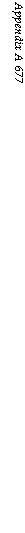Efficiency Calculation (Calculation A.1.2.1)

 S. No Losses GCV NCV 1A Dry gas losses WgXCpgX^-^XlOO^ WsxCP8(T8-ta)Xl00o/ GCV NCV 11.832 X 0.245 X 115 X 100 0. . Io b./U/o 5850 11.832 X 0.245 X 115 X 100 0. . /o b. y//o 5580 IB Moisture losses (9H2 + m) X (Hs — ha) X 100 0. Jo GCV 0.4618 X 633.2 X 100 0. . …. /o b. UU/o 5850 = 0 1C Humidity losses WmXCp(Tg-ta)Xl00o/ WmXCp(Tg-ta)Xl00 o/ GCV NCV 0.144 X 0.48 X 115 X 100 0. . ., ,0. —————————————— /0 — U. loo /0 5850 _ 0.144 X 0.48 X 115 X 100 0/ _ Q mo/ 5580 1. Stack losses = 1A +1B +1C = 10.83% = 1A +1B +1C = 6.11% 2. Radiation losses = 0.35% GCV — 0.35 X % — 0.37% NCV 3. Unburnt losses = 4.0% — 4 X GCV % — 4 x 5850 % — 4.20% NCV 5580 4. Unaccountable Losses = 0.5% — 0.5 X GCV %- 0.5 X 5850 %- 0.52% NCV 5580 5. Manufacturer Margin = 1.0% — 1 X GCV % — l x 5850 % — 1.05% NCV 5580 Total losses Sum of 1-5 = 16.68% Sum of 1-5 = 12.25% Gross thermal efficiency = 100 — total losses = 100 — 18.44 = 83.32% = 100 — total losses = 100 — 13.74 = 87.75%
 678 Appendix A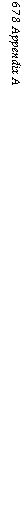TABLE A15

Total Air and Gas Weights (Calculation A.1.2.3)

 At Exit of Total Air Weight (kg/h) 8705 X Total Gas Weight (kg/h) 8705 X Total Air Weight (lb/h) 19,203 X Total Gas Weight (lb/h) 19,203 x Total Air weight (kg/s) 2.42 X Total Gas Weight (kg/s) 2.42 X Furnace Boiler bank Economizer Airheater Net induced draft fan 10.79 = 93,930 11.64 = 101,325 12.05 = 104,895 12.29 = 106,985 12.44 = 108,290 12.69 = 110, 465 10.79 = 207,200 11.64 = 223,520 12.05 = 231,400 12.29 = 236,005 12.44 = 238,885 12.69 = 243,685 10.79 = 26.11 11.64 = 28.17 12.05 = 29.16 12.29 = 29.74 12.44 = 30.10 12.69 = 30.71

Calculation A.1.2.2

Approximate estimation of efficiency on NCV from GCV Without doing a detailed calculation for efficiency on NCV, a nearly correct figure can be obtained from the efficiency of GCV by multiplying by the GCV/NCV ratio.

In the previous example this works out to be 83.32 X (5850/5580) = 87.35.

Calculation A.1.2.3

To calculate the fuel input into the boiler and the total air and gas weights. Refer to Table A15.

Heat duty

Fuel fired:

Thermal efficiency X GCV 51.42

 0.8332 X 24.49 44.2 X 106 0.8332 X 5850 " 175.5 X 1062.52 kg/s 9,068 kg/h or = 20,003 lb/h

0.8332 X 10,530 Fuel burnt = fuelfired X (1 — % unburnt loss/100)

= 2.52(1 — 4/100) = 2.42 kg/s = 9,068 X (1 — 4/100) = 8,705 kg/h = 20,003 (1 — 4/100) = 19,203 lb/h

A.1.3 Firing Equipment Selection

Calculation A.1.3.1

Selection of firing equipment.

Fuel fired by (rounding) is 9,070 kg/h or 20,000 lb/h, which has to be dealt with by a set of fuel feeders and grate. Since the fuel is coal, the dumping and reciprocating grates are unsuitable and only the spreader stoker is the choice.

Taking a nominal throughput of 2.5 tph or 5000 lb/h for each mechanical feeder, four feeders are adequate. Considering that one feeder may be down for maintenance, it is desirable to adopt a five-feeder configuration.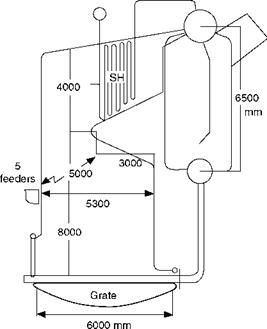FIGURE A2

Furnace and firing equipment.

This results in a furnace width of ~5 m (16.4 ft) to accommodate the five feeders in the front wall (Figure A2).

Many times the grates change from single to dual drive at 4.87 m (16 ft.). Therefore, it is prudent to limit the grate to a width of 4.87 m (16 ft).

For calculating the grate area, the permissible grate heat release rate has to be used consistent with the requirement of accommodating the superheater within the furnace arch depth. The final SH of only 452°C (750°F) means this can be accommodated in ~3 m and accordingly the depth of nose can be adopted as 3 m. The furnace depth can be kept at

5.3 m (17.38 in.) for a proper volumetric release rate.

Considering a grate of 4.87 m width X 5.543 m effective length, that is, 27 m2 (16 X 18.18 = 291 ft2), the grate heat release rate works out to be

• 51.42/27 = 1.9 MWth/m2

• 44.2 X 106/27.0 = 1,637,040 kcal/m2 h

• 175.5 X 106/291 = 603,090 Btu/ft2 h

This is within the permissible limits. See Section 11.4.1.2 for design aspects of stoker firing.

The actual length of stoker between the shaft centers is 457 mm (18 in.) more than the effective length, which makes the grate 4.87 m wide X 6.0 m deep (16 ft X 19.68 ft).

A.1.4 Preliminary Furnace Calculations Calculation A.1.4.1

To calculate the furnace volumetric heat release rate, residence time, and average gas velocity. Refer to Table A16.

TABLE A16

Furnace Volumetric Rate, Residence Time, and Gas Velocity (Calculation A.1.4.1)

 Metric and SI Units FPS Units Remarks Total furnace volume (12 X 2.3 + (8 — 1.73) X 3 + 1.73 X 3 X 1/2) X 5 =245 m3 = 8652 ft3 Sidewall area X furnace width Total heat input 9068 X 5850 = 53.048 Mkcal/h 2.52 X 24.49 = 61.71 MW 20,003 X 10,530 = 210.9 M Btu/h Fuel fired X GCV Volumetric heat release 216,550 kcal/m3/h 61.71 X 24,375 Btu/ft3/h Rate 1000/245 = 252 kW/m3 Volume up to center of 222 m3 7839 Total volume — 2.3 Exit plane X 2 X 5 = 245 — 23 = 222 m3 Weight of flue gas 101,325 kg/h 223,520 lb/h Furnace exit gas 950°C 1742°F Assumed Temperature Specific volume at 0.759 X (950 + 273)/273 = 3.4 12.16 X (1742 Furnace exit gas G /k 3m/ + 460)/492 Temperature = 54.42 ft3/lb Flue gas volume per 95.7 m3/s 3380 ft3/s Second Residence time 222/95.7 = 2.32 s 7839/3380 = 2.32 s Up to center of exit plane Average furnace area 222/10 = 22.2 m2 239 ft2 Volume/heat up to exit plane Average velocity 95.7/22.2 = 4.31 m/s 3380/39 = 14.1 ft/s

On the basis of Figure A2, calculations for different furnace parameters are done in Table A16.

A.1.5 Draft Plant Calculations Calculation A.1.5.1

Sizing of forced draft (FD) fan.

Sizing of fan involves calculating the capacity, head, and temperature at the MCR condi­tion, and adding suitable test block margins. Test block margins depend on the class of fan (performance tolerances), size of boiler, accuracy of determination of losses, etc. For industrial boilers of about this size, the margins considered here are normal. Altitude cor­rection is also required.

Forced draft fan is required to provide the necessary combustion air to the furnace after over­coming the resistances of the AH, grate, ducting, and air flow measurement device.

The site altitude is 305 m (1000 ft), and altitude correction factor is 1.04.

The combustion air quantity from Calculation A.2.2.3 is 93,930 kg/h or 26.11 kg/s or 207,200 lb/h.

Converting the weight into volume, we get

26.11 X 0.773 X 273 + 25 X 1.04 = 22.90 m3/s 273

273 + 25

= 93,930 X 0.773 X —3——————— 5 X 1.04 = 82,440 m3/h at 25°C

273

= 207,200 x 12.39 x 460 + 77 X 1.04 = 48,568 ft3/min at 77°F 60 460 + 32

Pressure drop across the AH = 490 Pa or 50 mm or 2.0 in. wg Ducts = 245 Pa or 25 mm or 1.0 in. wg Aerofoil = 118 Pa or 12 mm or 0.5 in. wg Undergrate pressure = 490 Pa or 50 mm or 2.0 in. wg = 1343 Pa or 137 mm or 5.5 in. wg

After altitude correction of 1.04 = 1397 Pa or 142.5 mm or 5.72 in. wg.

In SI units.

• MCR duty of the FD fan is 22.9 m3/s at 1343 Pa wg and 25°C.

• Design duty is 22.9 X (273 + 45)/(273 + 25) X 1.2 at (1343 — 490) X (1.44 + 490) X

1.4 and 45°C = 29.32 m3/s at 1787 Pa wg and 45°C.

The design duty is calculated by adding 20% margin on volume and 44%, being square of

1.2 On the variable static head and 20°C on the temperature (or the highest ambient temperature whichever is higher). Undergrate pressure is constant here for which the design head has to be suitably calculated as shown in the preceding text.

In MKS units.

• MCR duty of the FD fan is 82,440 m3/h at 142.5 mm wg and 25°C.

• Design duty is 82,440 X (273 + 45)/(273 + 25) X 1.2 at (137 — 50) X (1.44 + 50) X 1.04

And 45°C = 105,567 m3/h at 182.3 mm wg and 45°C.

In FPS units.

• MCR duty of the FD fan is 48,568 ft3/m at 5.72 in. wg and 77°F.

• Design duty is 48,568 X (460 + 111)/(460 + 77) X 1.2 at (5.5 — 2.0) X (1.44 + 2.0) X

1.4 at 97°F = 61,972 ft3/m at 7.32 in. wg and 111°F.

Calculation A.1.5.2

Sizing of induced draft (ID) fan.

Anticipated weights of the exhaust gases at the ID fan inlet are tabulated in Calculation A.1.2.3 and Table A15.

Flue gas quantity at ID fan inlet is 110,465 kg/h or 243,685 lb/h at 135°C or 275°F assum­ing a small temperature drop of 5°C (10°F) between AH and ID fan in its passage through the electrostatic precipitator (ESP).

Specific volume at ID fan inlet is taken from Calculation A.1.1.8.

Converting into volume with altitude correction, we get

 273 + 135 27330.71 X 0.761 X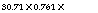X 1.04 = 36.32 m3/s at 135°C

 273 + 135 273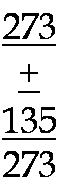110,465 X 0.761 X 60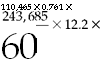460 + 275 460 + 32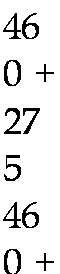X 1.04 = 130,660 m3 /h at 135°C

X 1.04 = 76982 ft3/min at 135°C

Draft loss account: furnace draft is 59 Pa or —6 mm or 0.25 in. wg

Loss in SH is 59 Pa or —6 mm or 0.25 in. wg

Loss in boiler bank (BB) is 490 Pa or —50 mm or 2.0 in. wg

Loss in economizer (ECON) is 372 Pa or —38 mm or 1.5 in. wg

Loss in AH is 490 Pa or —50 mm or 2.0 in. wg

Loss in ESP is 196 Pa or —20 mm or 0.8 in. wg

Loss in ducts is 196 Pa or —20 mm or 0.8 in. wg

Total losses is 1862 or -190 mm or 7.6 in. wg

After altitude correction is 1940 or -198 mm or 7.9 in. wg

In SI units.

• MCR duty of the ID fan is 36.62 m3/s at 1940 Pa wg and 135°C.

• Design duty with 20% margin is 43.94 X (273 + 155)/(273 + 135) m3/h at (1862 — 59) X (1.44 + 59) X 1.04 Pa and 155°C = 46.1 m3/s at 2762 Pa wg and 155°C.

The test block margins are similar to FD fan except for the margin on temperature, which is taken as 20°C or 35°F.

In MKS units.

• MCR duty of the ID fan is 130,660 m3/h at 198 mm wg and 135°C.

• Design duty with 20% margin is 156,792 X (273 + 155)/(273 + 135) m3/h at (190 — 6) X (1.44 + 6) X 1.04°C and 155°C = 164,478 m3/h at 282 mm wg and 155°C.

In FPS units.

• MCR duty of the ID fan is 76,982 ft3/m/h at 7.9 wg and 275°F.

• Design duty is 76,982 X (460 + 310)/(460 + 275) X 1.2 at (7.6 — 0.25) X (1.44 + 0.25) X

1.4 at 310°F = 96,778 ft3/m at 11.27 in. wg and 310°F.

Calculation A.1.5.3

Sizing of secondary air (SA) fan.

Sizing of SA fan varies from manufacturer to manufacturer depending on the firing equipment and the design practice. For a spreader stoker for burning coal, it is usual to

Provide a fan that gives 20% combustion air to the furnace at a pressure of 635 mm or 6225 Pa or 25 in. wg. Starting from the design duty of FD fan in calculation A.1.5.1,

SA fan design condition = 29.32 X 0.2 = 5.864 m3/s at 6225 Pa and 35° C

= 105,567 X 0.2 = 21,113 m3/h at 635 mm w. g. and 35° C = 61,972 X 0.2 = 12,394 ft3/m at 25 in. w. g. and 97° F

Calculation A.1.5.4

Sizing of ESP. Refer Table A17.

Sizing practice varies among the manufacturers, depending on the clients’ specifica­tions, experience, and practice. The following procedure may be considered.

Primarily, an ESP is sized to give a certain committed dust concentration at the outlet for a definite inlet dust loading. Any variation in the inlet load gets reflected at the outlet in a sympathetic manner. This is in sharp contrast to the fabric filter (FF), which is insensitive to the inlet dust loading and provides a constant outlet concentration.

Estimating the inlet dust concentration is the crucial part. The outlet concentration is specified in any region by the local pollution control authorities. Let us consider the permissible dust concentration as 100 mg/N m3 and calculate the inlet dust loading as done in Table A17.

The ESP construction note will be as follows:

In SI units.

• MCR duty is 23.79 N m3/s at 140°C with dust loading of 5.05 mg/N m3 at inlet and 100 mg/N m3 at outlet with gas draft loss limited to 196 Pa wg.

• Design duty 36.0 N m3/h at 160°C with dust loading of 3.33 mg/N m3 at inlet and 100 mg/N m3 at outlet with draft loss limited to 284 Pa wg.

In MKS units.

• MCR duty is 85,892 N m3/h at 140°C with dust loading of 5.15 g/N m3 at inlet and 100 mg/N m3 at outlet with gas draft loss limited to 20 mm wg.

• Design duty is 103,740 N m3/h at 160°C with dust loading of 3.4 mg/N m3 at inlet and 100 mg/N m3 at outlet with draft loss limited to 28 mm wg.

In FPS units.

• MCR duty is 50,433 N ft3/m at 284°F with a dust loading of 2.25 gr/N ft3 at inlet and 0.0437 gr/N ft3 at outlet with draft loss limited to 0.8 in. wg.

• Design duty is 60,520 N ft3/m at 320°F with dust loading of 2.25 gr/N ft3 at inlet

And 0.0437 gr/N ft3 at outlet with draft loss limited to 1.15 in. wg.

In this example the same dust loading is considered for both MCR and design condi­tions, which means a margin for 20% increase in dust elutriation due to a possible higher ash coal. There can be different methods of applying the margins, which can result in bet­ter economy with adequate latitude.

TABLE A17

Sizing of Electrostatic Precipitators (Calculation A.1.5.4)

 Parameter SI Units Metric Units FPS Units Remarks Fuel fired 2.52 kg/s 9070 kg/h 20,000 lb/h Ash input 0.38 kg/s 1360 kg/h 3,000 lb/h Ash forms 15% of coal Ash elutriated 0.15 kg/s 545 kg/h 1,200 lb/h Ash split is 30:70%::fly: bed ash in spreader stokers. For design 40% fly ash is taken Ash at ESP inlet 0.15 X 0.9 X 0.9 = 545 X 0.9 X 0.9 1,200 X 0.9 X 0.9 Assume 10% 0.12 kg/s = 441 kg/h = 972 lb/h Precipitation in BB and AH hoppers Gas weight at AH 30.1 kg/s 108,290 kg/h 238,885 lb/h From Calculation A.1.2.3 Exit Specific volume at 0.76 N m3/kg 0.76 N m3/kg 12.18 N ft3/lb From Calculation A.1.8 NTP Specific volume at 1.15 A m3/kg 0.76 X (273 12.18 X (460 AH outlet + 140)/273 = 1.15 A m3/kg + 284)/460 = 19.7 ft3/lb Gas volume at ESP 30.1 X 0.76 X 1.04 108,290 X 0.76 238,885 X 12.18 X At NTP conditions with Inlet = 23.79 N m3/s X 1.04 = 85,592 N m3/h 1.04 = 3,026,003 N ft3/h or 50,433 N ft3/m Altitude correction Gas volume at ESP 30.1 X 1.15 X 1.04 108,290 X 1.15 238,885 X 19.7 X At ESP inlet temperature Inlet = 36.0 A m3/s X 1.04 = 129,514 A m3/h 1.04 = 4,894,276 A ft3/h or 81,571 N ft3/m 140°C/84°F ESP inlet dust (0.12 X 1000)/23.79 (441 X 1000)/ (972 X 7000)/ Concentration = 5.05 g/N m3 85,592 = 5.15 g/N m3 3,026,003 = 2.25 gr/N ft3 ESP inlet dust (0.12 X 1000)/36 = (441 X 1000)/ (972 X 7000)/ Concentration 3.33 g/N m3 129,514 = 3.40 G/A m3 4,894,276 = 1.39 g/A ft3 Outlet 100 mg/N m3 100 mg/N m3 0.0437 gr/S ft3 Assumed as local limit Concentration Collection 1 — (100/(5.15 Efficiency X 1000))100 = 98.06% Design volume 23.79 X 1.2 = 28.55 85892 X 1.2 50,433 X 1.2 = Same margin as ID fan N m3/s = 103,074 N m3/h 60,520 N ft3/m Design temperature 160°C 140 + 20 = 160°C 284 + 36 = 320°F Same margin as ID fan Permissible draft 196 and 284 Pa 20 and 29 mm 0.8 in. and 1.15 in. At MCR and design Loss (196 X 1.44) wg (20 X 1.44) wg (0.8 X 1.44) wg Conditions

Calculation A.1.5.5

Sizing of air ducts. Refer Table A18.

Starting from the total air weights calculated in Calculation A.1.2.3 and Table A15, the minimum duct sizes based on the permissible gas velocities are worked out in Table A18.

Calculation A.1.5.6

Sizing of gas ducts (flues). Refer Table A19.

Starting from the total gas weights in Calculation A.1.2.3 and Table A.15, the minimum five sizes based on permissible velocities are worked out in Table A19.

TABLE A18

Sizing of Air Ducts (Calculation A.1.5.5)

 Metric and SI Units FPS Units Parameter FD Discharge Hot Air Duct from AH FD Discharge Hot Air Duct from AH Remarks Air weight at MCR 93,930 kg/h 26.1 kg/s 93,900 kg/h 26.1 kg/s 207,200 lb/h 207,200 lb/h Operating 25°C 100°C 77°F 212°F Temperature Specific volume at 0.773 X (298/273) 0.773 X (373/273) 12.39 X (537/492) 12.39 X (672/492) Operating = 0.844 A m3/kg = 1.056 A m3/kg = 13.52 A ft3/lb = 16.92 A ft/lb Temperature Flow (altitude corrected) 82,450 A m3/h 22.90 A m3/s 103,155 A m3/h 28.65 A m3/s 48,560 A ft3/m 60,780 A ft3/m X 1.04 Velocity Permissible 11 m/s 15 m/s 2200 ft/min 3000 ft/min Refer to Section 7.3.1.1, Table 7.5 Minimum area 2.08 m2 1.91 m2 22.07 20.26

A.1.6 Sizing of Valves and Mountings Calculation A.1.6.1

To determine the boiler design pressure. Refer to Table A20.

Step 1. Calculate the drum operating pressure by adding the various pressure drops to the superheater outlet pressure (SOP). Drop in SH is calculated but others are assumed based on experience. All pressure losses can be calculated at the contract stage after all valves are ordered and detailed drawings are prepared. For most boilers, such calculations are not warranted.

The SH pressure drop is assumed to be 2.75 bar, 2.8 kg/cm2, or 39.8 psig (Figure A3). With superheater outlet temperature (SOT) < 425°C it is assumed that there is a spray attemperator at the outlet of SH.

Step 2. Add 5% to the drum operating pressure to arrive at the first safety valve lift­ing pressure (SVLP). It is assumed that the blowdown of the safety valve [(lifting pressure minus reseating pressure)/lifting pressure] is <5%, which is usually the case for safety valves used in industrial boilers. For the second SVLP, usually there is a stepping of a minimum of 0.34 bar, 0.35 kg/cm2, or 5 psig.

Boiler design pressure is the highest SVLP, which is usually the second SVLP unless the boiler demands more SVs. Calculations are performed as per Table A20.

Calculation A.1.6.2

Sizing of safety valves. Refer to Table A21.

The following guidelines are to be kept in mind while making a selection of SVs for a boiler.

• SVs are the most sophisticated, expensive, and delicate valves and their number should be kept to a minimum.

TABLE A19

 Appendix A 687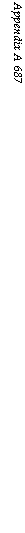Sizing of Gas Ducts (Flues) (Calculation A.1.5.6)

 Metric and SI Units FPS Units Boiler Bank Exit Economizer Exit AH/ESP Exit ID Fan Discharge Boiler Bank Exit Economizer Exit AH/ESP Exit ID Fan Discharge Remarks Gas at MCR 104,895 29.14 106,985 29.71 108,290 30.08 110,465 30.68 231,400 236,005 238,885 243,635 Kg/h and lb/hkg/s Temperature 400°C 200°C 140°C 135°C 752°F 392°F 284°F 275°F Temperature Assumed Specific volume \$ X 673/273 \$ X 473/273 \$ X 413/273 \$ X 408/273 Ј X 1212/492 Ј X 852/492 Ј x 744/460 Ј X 735/460 \$ = 0.76 and (m3/kg and ft3/lb) = 1.87 = 1.316 = 1.15 = 1.136 = 30.00 = 21.1 cf/lb = 19.69 = 19.46 Ј = 12.18 specific volume at NTP Flow (m3/ s and ft3/m) 56.67 40.67 35.97 35.93 120,338 86,295 81,527 82,196 Altitude Corrected (1.04) Velocity range 12.5-15 12.5-15 12.5-15 9-14 2500-3000 2500-3000 2500-3000 1800-2800 M/sandft/min Velocity 14 m/s 14 m/s 14 m/s 12 m/s 2750 ft/min 2750 ft/min 2750 ft/min 2350 ft / min Refer to Section 7.3.1.1, Table 7.5 Minimum area 4.05 m2 2.90 m2 2.57 m2 3.0 m2 43.75 ft2 31.38 ft2 29.64 ft2 35.0 ft2

Boiler Design Pressure Calculation (Calculation A.1.6.1)

 Bar MPa Kg/cm2 g Psig Superheater outlet pressure (from Calculation 1.1.1) 70 7.0 70.38 1000 + Pressure drop in main stem stop value + 0.7 10 Nonreturn valve + Pressure drop in main header 0.35 5 + Spray attemperator loss 0.35 5 + Pressure drop in superheater bank 2.81 40 Drum operating pressure 74.18 7.418 74.6 1060 First SVLP: drum pressure + 5% 77.9 7.79 78.3 1113 Second SVLP: first SVLP + stepping 78.4 7.84 78.8 1120 Boiler design pressure 78.4 7.84 78.8 1120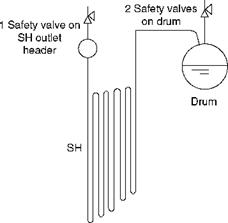FIGURE A3

Superheater.

• The total relieving capacity of the safety valves on the drum and the SH should equal or exceed the boiler peak duty evaporation in drum-type boilers. Likewise, they should equal or preferably exceed the maximum RH flow.

• SVs on the SH have to deal with SH steam and hence are more expensive and main­tenance-prone. SVs should be sized for not less than 20% steam flow to provide enough flow to prevent overheating of SH tubes.

• Steam drums should always have two SVs as a minimum.

In the current example, the SVs have to be sized as in Table A21. For most industrial boilers, three SVs are adequate—one on SH and two on drum.

Rows 1,5,6,7, and 8 form the specification of SVs for purchase.

Calculation A.1.6.3

Sizing of main steam stop valve (MSSV) and nonreturn valves (NRV). Refer to Table A22.

NRV is required only when two or more units operate in parallel to feed a common steam distribution header (CSDH). The nominal bore (Nb) of the NRV is the same as the MSSV.

TABLE A21

Sizing of Safety Valves (Calculation A.1.6.2)

 Size Parameter Superheater Drum 1 Drum 2 Remarks 1 No. off 1 1 1 Total three safety valves 2 Capacity (%) >20% 1212 ~40% ~40% >100% 3 Operating 70.38 kg/ 1000 74.6 kg/ 1060 74.6 kg/ 1060 See previous calculation Pressure Cm2 g Psig Cm2 g Psig Cm2 g Psig For operating pressure and SVLPs 4 Operating 400°C 752°F Sat Sat Sat Sat Temperature (290°C) (553°F) (290°C) (553°F) 5 Flow >13,600 >30,000 ~27,200 ~ 60,000 ~27,200 ~60000 Total flow should exceed Kg/h Lb/h Kg/h Lb/h Kg/h Lb/h Boiler evaporation 6 SVLP 73.9 kg/ Cm2 g 1050 78.3 kg/ Cm2 g 1113 78.8 kg/ Cm2 g 1120 1.05 X operating pressure stepping for drum safety valves 7 Design 425°C 797°F Sat Sat Sat Sat 25°C/45°F attemperation Temperature (293.5°C) (559°F) (293.5°C) (559°F) At SH outlet raises design temperature 8 Material of construction MC MC MC MC MC MC MC is medium carbon steel. Selected as steam temperature is low
 TABLE A22 Sizing of Main Steam Piping (Calculation A.1.6.3) Parameter SI Units Metric Units FPS Units Remarks Steam flow 18.895 kg/s 68,020 kg/h 150,000 lb/h (Calculation 1.1) Design 70 bar/7.0 MPa 70.38 kg/cm2 g 1000 psig Pressure/SOP Design 400°C + 5°C 400°C + 5°C 752°C + 9°C After attemperation Temperature/SOT = 405°C = 405°C = 761°F Specific volume of 0.039 m3/kg 0.039 m3/kg 0.649 ft3/lb Steam Volume of steam 0.737 m3/s 0.737 m3/s 27.04 ft3 /s Ws X specific volume/3600 Flow per second Steam velocity 45 m/s 45 m/s 150 ft/s Refer to Table 7.4 Area required 0.0163 m2 0.0163 m2 0.18 ft2 As = volume flow/steam velocity Pipe inner diameter 0.144 m or 0.144 m or 0.48 ft X 12 |4xAs 144 mm 144 mm = 5.75 in. V / Nominal bore 150 mm 150 mm 6 in. 100 mm bore will give velocity Selected Greater than permissible Nominal steam 41.7 mPs 41.7 mps 137.8 ft/s Volume per second per area Velocity

These valves are the largest and operate at the highest temperature. Their selection has to be optimal, both from cost and from O&M considerations.

Main steam (MS) piping size is the size of MSSV and NRV, which may be many times

A size lower than the main steam outlet header that needs to support several valves and

Cannot have the same high steam velocity as the MS pipe.

Permissible steam velocities for steam range from 30 to 60 m/s (100-200 ft/s) lower veloc­ities for lower pressures and temperatures. Calculations are started with a mean velocity of 45 m/s (150 ft/s).

TABLE A23

Sizing of Feed Line (Calculation A.1.6.3)

 SI Units Metric Units FPS Units Remarks Feed water flow 18.895 kg/s 68,020 kg/h 150,000 lb/h Design pressure of piping 95.86 bar/9.586 96.7 kg/cm2 g 1380 psig ~1.3 X drum operating pressure Design temperature/feed MPa 105°C 105°C 221°F Water temperature Specific volume of water 0.001048 0.001048 0.01677 Take specific volume of saturated M3/kg M3/kg Ft3/lb Water Volume of water flow 0.0198 m3/s 71.285 m3/h 2515.5 ft3/h Ww X specific volume/3600 Water velocity adopted 2.5 m/s 0.0198 m3/s 2.5 m/s 0.6987 ft3/s 8 ft/s Refer to Table 7.4 Area required 0.0079 m2 0.0079 m2 0.0852 ft2 As = volume flow/steam velocity Pipe id 0.100 m 0.100 m 0.33 ft X 12 |4xAw Or 100 mm Or 100 mm = 3.95 in. V n Nominal bore selected 100 mm 100 mm 4 in. 76 mm (3 in.) bore will give Nominal water velocity 2.5 mps 2.5 mps 8 ft/s Greater than permissible velocity Volume per second per area

The main steam outlet header should be chosen as 200 mm (8 in. NB) while the main steam piping should be 150 mm (6 in. NB) with the MSSV and NRV (if applicable) also of 150 mm (6 in. NB) size.

Calculation A.1.6.4

Sizing of feed check valve and feed water piping. Refer to Table A23.

The range of permissible velocities of water in feed piping is between 2 and 3 m/s. The design pressure of feed piping from the boiler feed pump (BFP) to the upstream flange of feed control valve is the no-load pump pressure of the BFP. It is important for pressure calculation and not for sizing of water piping, as the specific volume is not very dependent on water pressure, unlike steam. For a first approximation the no-load pump pressure (NLPP) can be taken as 1.3 times the drum pressure (Table A23).

A.1.7 Sizing of Pumps Calculation A.1.7.1

Sizing of boiler feed pump. Refer to Table A24. Refer to Section 2.5.8.3 for BFP sizing.

As a BFP is required to feed water to the drum even when the drum safety valves are blowing to maintain the drum level, the highest SVLP becomes the basis for the estimation of BFP discharge pressure. Table A24 leads us to the BFP discharge pressure.

For sizing the MCR flow of the BFP, continuous blowdown (CBD) flow has to be added to the feed water flow. Normally a 3% CBD is considered adequate. Since the BFP is provided with a flooded suction, many times the static head available due to the elevation of the deaerator is subtracted from the total head calculated earlier. The head at the pump suct­ion, which is the net positive suction head available (NPSHA), is the static head from the trip limit at the bottom of the deaerator tank to the center line of the pump suction minus the friction loss in the BFP suction line (Figure A4).

TABLE A24

 FIGURE A4 Schematic of boiler feed pump and feed line.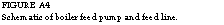Sizing of Boiler Feed Pumps (Calculation A.1.7.1)

 Pressure Loss Account Kg/cm2 g Bar MPa Psig Remarks Second SVLP/boiler 78.80 78.3 7.83 1120 Design pressure + loss in -0.35 -5 Economizer + loss in NRV -0.35 -5 + loss in piping 1.0 -15 + loss in control 2.1 -30 Valve + loss in flow -0.35 -5 Measurement + static head 1.83 26 Height between centers of drum and BFPs assumed to be 18.3 m (60 ft). Density correction is ignored + loss in HP heater 0 0 No heater in this example BFPs discharge 84.78 84.17 8.417 1206 Pressure Design margin 86.92 86.27 8.627 1240 At 2.5% Converting to 888.5 m 888.5 m 888.5 m 2912 ft Pressure X specific volume X 10,000 Head—MCR Form and pressure X specific volume X 144 for ft Design 910.7 m 910.7 m 910.7 m 2985 ft Take specific volume from earlier calculation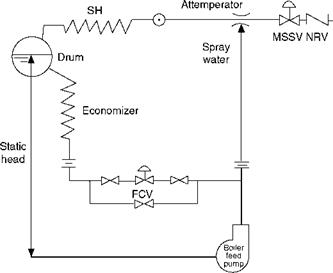Often this small adjustment is ignored intentionally and added to the overall safety margin.

Test block margin for flow is 10%. Assuming there are 2 X 100% BFPs, one working and one standby, the pump specifications work out as follows:

In SI units.

• MCR condition: 0.0198 X 1.03 is 0.0204 m3/s of flow at 105°C and 888.5 m

• Design condition: 0.0204 X 1.10 is 0.0224 m3/s of flow at 105°C and 910.7 m

In MKS units.

• MCR condition: 71.28 X 1.03 is 73.42 m3/h of flow at 105°C and 888.5 m

• Design condition: 73.42 X 1.10 is 80.76 m3/h of flow at 105°C and 910.7 m

In FPS units.

• MCR condition: 2515 X 103 is 2590 ft3/h of flow at 221°F and 2912 ft of water

• Design condition: 2590 X 1.10 is 2850 ft3/h of flow at 221°F and 2985 ft of water

A.1.8 Sizing of Control Valves Calculation A.1.8.1

Sizing of control valves.

There are only two types of control valves in boiler, namely, the feed and attemperator control valves.

Feed control valve. The main control valve is classically one size smaller than the feed pip­ing for good controllability. In the current example, the feed line is 100 mm (4 in. NB) and the FCV is 80 mm (3 in. NB).

When start-up and low load control valves are to be provided for a 40-50% load, they are sized for a velocity of 3-4 m/s (nominal).

The flow conditions are taken from the BFP on the upstream side. The valve rating should be adequate for the NLPP.

Attemperator control valve. A spray attemperator at the discharge of SH is assumed, which would desuperheat the steam from 425° to 400°C (797-752°F), with spray water provided from the BFP. The following heat balance around the attemperator gives the spray water quantity. Heat in input streams of spray water and steam is equal to heat in attemperated steam.

Spray water at 105°C + steam at SH outlet at 425°C = final steam of 68.02 tph at 400°C at 70.38 kg/cm2

105 X SW + 770.0 X (68,020 — SW) = 754 X 68,020

NT47 68,020 X (770.0 — 754) „ „

SW = ——————————————————————— — — = 1,636 kg/h

770 — 105 ‘S’

Attemperator flow is ~1650 kg/h. But the spray valve should be sized for twice the calcu­lated flow, keeping in mind the possibility of slagging and fouling (Figure A5).

 Spray water I at 105 °C 1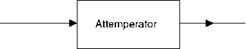Steam at 425 °C Steam at 400 °C

FIGURE A5

Schematic of spray attemperator at superheater outlet.

A.1.9 Sizing of Drives Calculation A.1.9.1

Sizing of drive motors for fans. Refer Table A25.

Fan hp can be calculated by the formula in Section 8.2.8, reproduced as follows:

Fan power (hp) = kVH/nc

Where k = compressibility factor

V = inlet volume in cubic meters per hour (ft3/m) H = pressure rise across fan (mm wg) (in.) c = constant 2743 (63.54) n = fan static efficiency

Compressibility factor for air or 1.0 1.05 1.1 1.15

Flue gas

Pressure ratio 1.0 0.98 0.97 0.95

Fan power in kW in SI units is given by the formula kW = kVH/nc where V is in m3/s, H in kPa, c = 1, n is % efficiency. It can also be expressed as kW = 0.1 X kVH/nc where H is in mbars.

In the present context the hp of all fans can be calculated as shown in Table A25, ignor­ing the compressibility factor.

Calculation A.1.9.2

Sizing of drive motors for pumps. Refer to Table A26.

WApv

Pump brake horsepower (Bhp) = ^n or

IGPM X head in ft of water X sp. gr at 60°F/30 in. wg 3300^

Where W = flow in lb/h

Ap = differential pressure in psi v = specific volume of liquid at the pump entry ft3/lb n = % pump efficiency/100

 Fan SI Units Metric Units FPS Units Remarks Forced Duty 29.32 m3/s at 105,567 m3/h at 61,972 ft3/m at Backward-bladed Draft fan 1.787 kPa and 45°C 182.3 mm wg and 45°C 7.32 ft wg and 111°F Fan chosen Hp calculation (105,567 X 182.3)/(2743 X 70) = 100.2 (61,972 X 7.32)/(63.54 X 70) = 102 Fan efficiency assumed 70% Compressibility factor ignored KW calculation (29.32 X 1.787)/ = 74.85 0.70 Motor hp 100.2 X 1.15 = 115.2 102 X 1.1.5 = 117.3 15% margin over fan hp Motor kW 74.85 X 1.15 = 86 0.746 X 115.2 = 86 0.746 X 117.3 = 87.5 Use 125 hp/94 kW standard motor Induced Duty 46.1 m3/s at 2.762 164,478 m3/h at 96,778 ft3/m at Backward-bladed Draft fan KPa and 155°C 282 mm wg and 155°C 11.27 ft wg and 310°F Fan chosen Hp calculation (164,478 X 282)/(2743 X 75) = 228 (96778 X 11.27)/ (63.54 X 75) = 229 Fan efficiency assumed 75% KW calculation (46.1 X 2.762)/0.75 = 169.76 Motor hp 228 X 1.15 = 263 229 X 1.15 = 263 15% margin over fan hp Motor kW 169.76 X 1.15 = 195.2 0.746 X 263 = 196 0.746 X 263 = 196 Use 270 hp/200 kW standard motor Secondary Duty 5864 m3/s at 21,113 m3/h at 12,394 ft3/m at 25 Radial-bladed fan Air fan 6.225 kPa and 45°C 635 mm wg and 35°C In. wg and 97°F Chosen Hp calculation (21,113 X 635)/(2743 X 60) = 81.5 (12,394 X 25)/ (63.54 X 60) = 81.3 Fan efficiency assumed 60% KW calculation (5.864 X 6.225)/ 60.8= 0.6 Motor hp 81.5 X 1.15 = 93.7 81.3 X 1.15 = 93.5 15% margin over fan hp Motor kW 60.8 X 1.15 = 70 0.746 X 93.7 = 69.9 = 70 0.746 X 93.5 = 69.7 = 70 Use 100 hp/75 kW standard motor

TABLE A26

Sizing of Boiler Feed Pump Drive (Calculation A.1.9.2)

 Parameter SI Units Metric Units FPS Units Remarks Design 0.0224 m3/s at 105°C 80.76 m3/h at 105°C 2850 ft3/h at 221°F and 1 ft3/h = 0.1037 IGPM Condition And 910.7 m And 910.7 m 2985 ft Pump hp (80.76 X 910.7 X (2850 X 0.1037 X 2985 X Specific gravity = 0.954 0.954)/(273 X 0.954)/(3300 X 0.72) = At 105°C 0.72) = 356 354 Pump kW (9.837 X 0.0224 X 910.7 X 0.954)/0.72 = 266 356 X 0.746 = 266 Motor 266 X 1.15 = 306 A margin of 15% over Rating The pump rating

In metric units pump Bhp = (Q x h x specific gravity)/273 x n where Q = discharge in cubic meters per hour h = head in meters n = pump efficiency in percentage

The efficiency of the feed pump is usually in the range of 70-85%.

In SI units pump (kW) = (9.837 x h x specific gravity)/273 x n where Q = discharge in cubic meters per hour H = head in meters n = pump efficiency in percentage

In this example, the Bhp of the feed pump can be worked out as shown in Table A26.